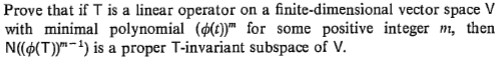# Prove that if T is a linear operator on a finite-dimensional vector space Vwith minimal polynomial (())" for some positive integerN((6(T))"-) is a proper T-invariant subspace of V.m, then

Question
1 viewshelp_outlineImage TranscriptioncloseProve that if T is a linear operator on a finite-dimensional vector space V with minimal polynomial (())" for some positive integer N((6(T))"-) is a proper T-invariant subspace of V. m, then fullscreen

### This question hasn't been answered yet.